## AI & Ruby: an introduction to neural networks

## Fundamentals of AI, machine learning and neural networks while building a neural network in Ruby

Currently, we find ourselves in the middle of the hype surrounding Artificial Intelligence (AI) and all its buzzwords.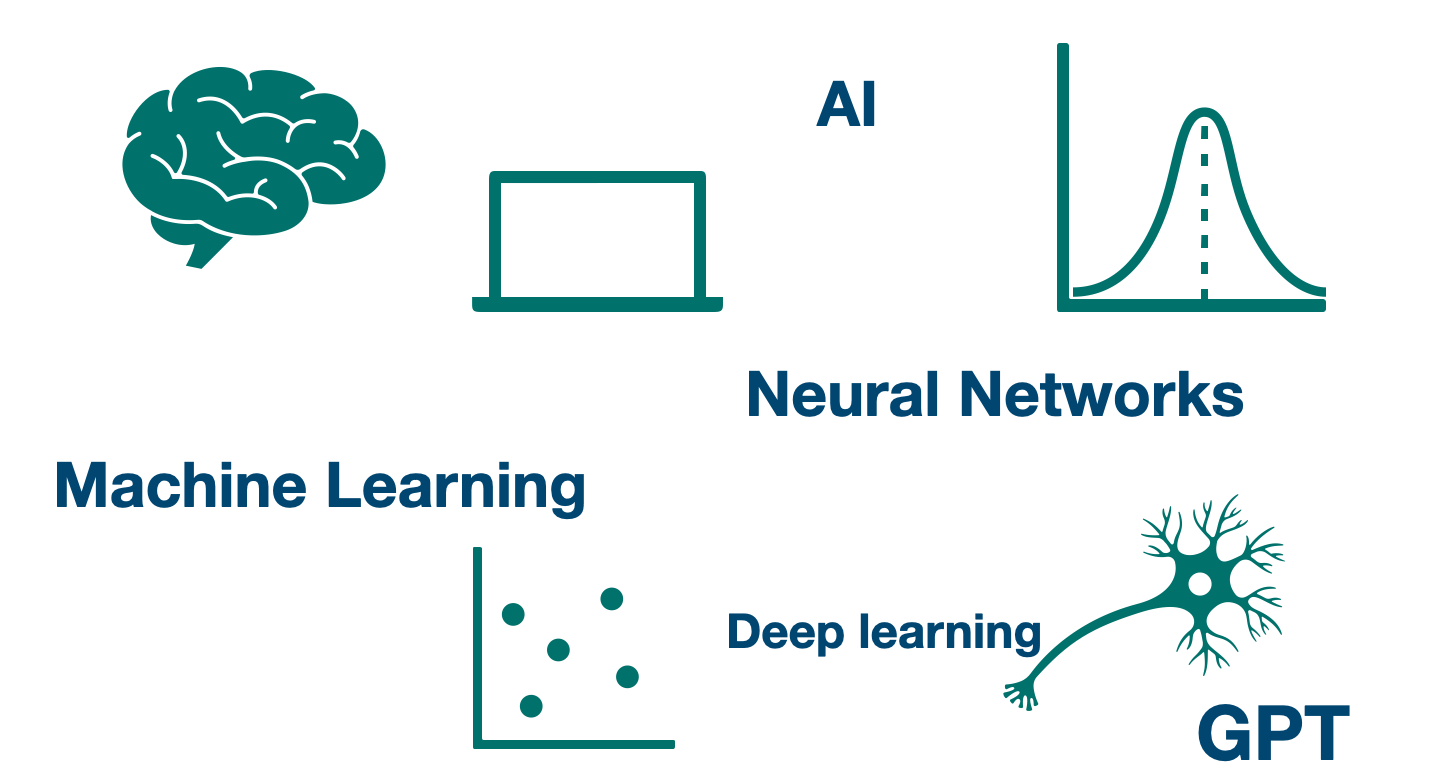It's natural to feel that terms related to AI can be complex and overwhelming to many individuals.

This article aims to demystify AI applications, delve into Machine Learning (ML) through the creation of a basic Artificial Neural Network (ANN) in Ruby, and shed light on the ever-present "GPT" phenomenon.

Join me on this enlightening journey!

## 🤖 An overview of AI

Artificial Intelligence (AI) is a branch of computer science focused on creating "intelligent machines" capable of performing tasks that simulate human intelligence.

The history of AI dates back to the 1950s, with the development of early AI programs and concepts.

Some notable AI milestones include the development of expert systems in the 1970s, the rise of machine learning in the 1990s, and the recent advancements in deep learning and neural networks.

AI has a wide range of real-world applications across various industries, including healthcare, finance, manufacturing, transportation, and entertainment. It is used in areas such as speech recognition, image classification, autonomous vehicles, virtual assistants, fraud detection, and recommendation systems.

### 🔵 AI algorithms

To address real-world problems, AI employs various algorithms designed to handle specific scenarios. Here are a few examples:

👉 Rule-based systems

These systems often rely on predefined rules to make decisions based on given conditions.

They are commonly used in domains such as medical diagnosis and fraud detection.

👉 Natural Language Processing

NLP (Natural Language Processing) plays a crucial role in bridging the gap between computers and human language.

Applications such as sentiment analysis and chatbots, including GPT (Generative Pre-trained Transformer), heavily rely on NLP techniques.

👉 Search engines

Search engines commonly employ AI techniques, including NLP (Natural Language Processing), used for tasks like tokenization and indexing.

Major search engines like Google Search and Microsoft Bing make use of these techniques to enhance their functionality.

👉 Machine Learning

Machine Learning (ML) is another vital component of AI that empowers AI systems to automatically learn and improve from experience or data.

It is widely applied in various domains, including pattern recognition, data prediction, and classification.

Now, let's delve into the realm of machine learning algorithms.

## 💡 An Overview of Machine Learning

Machine learning (ML) is a subfield of artificial intelligence (AI) that focuses on the development of algorithms and models that allow computer systems to learn and make predictions or decisions without being explicitly programmed.

It is based on the idea that machines can analyze and interpret complex patterns and data, and use them to improve their performance or behavior over time.

### 🔵 ML algorithms

Below there are several ML algorithms along with their real-world applications that reap the benefits of machine learning:

👉 Linear Regression

Linear Regression is a statistical method used to determine the optimal linear relationship between input variables and their corresponding outputs.

It finds extensive applications in fields such as economics and finance, enabling the prediction of house or stock prices and the estimation of sales revenue, among other uses.

👉 K-Nearest Neighbors

K-Nearest Neighbors (KNN) is an algorithm used for data classification, where the classification is based on the majority similarity among the "k nearest" neighbours.

Recommendation systems often leverage KNN to make personalized recommendations by identifying items or content with similar characteristics or behaviours.

👉 Neural Networks

Artificial Neural Networks (ANNs) are powerful deep learning models that aim to simulate the structure and functionality of the human brain.

They consist of interconnected layers of neurons that utilize mathematical activation functions to update weights based on proximity and learn from the data.

ANNs find extensive applications in various domains, including image recognition, where they excel in identifying and classifying objects within images. Additionally, ANNs are used for language translation tasks, leveraging their ability to process and understand textual data.

Furthermore, ANNs are extensively employed in conjunction with large language models (LLMs) and NLP techniques, particularly in the development of chatbot systems based on models like GPT (Generative Pre-trained Transformer).

These advanced neural network architectures enable chatbots to generate human-like responses and engage in natural language conversations with users.

Yes, we are talking about you, ChatGPT!

An overview of how AI, ML and neural networks are related: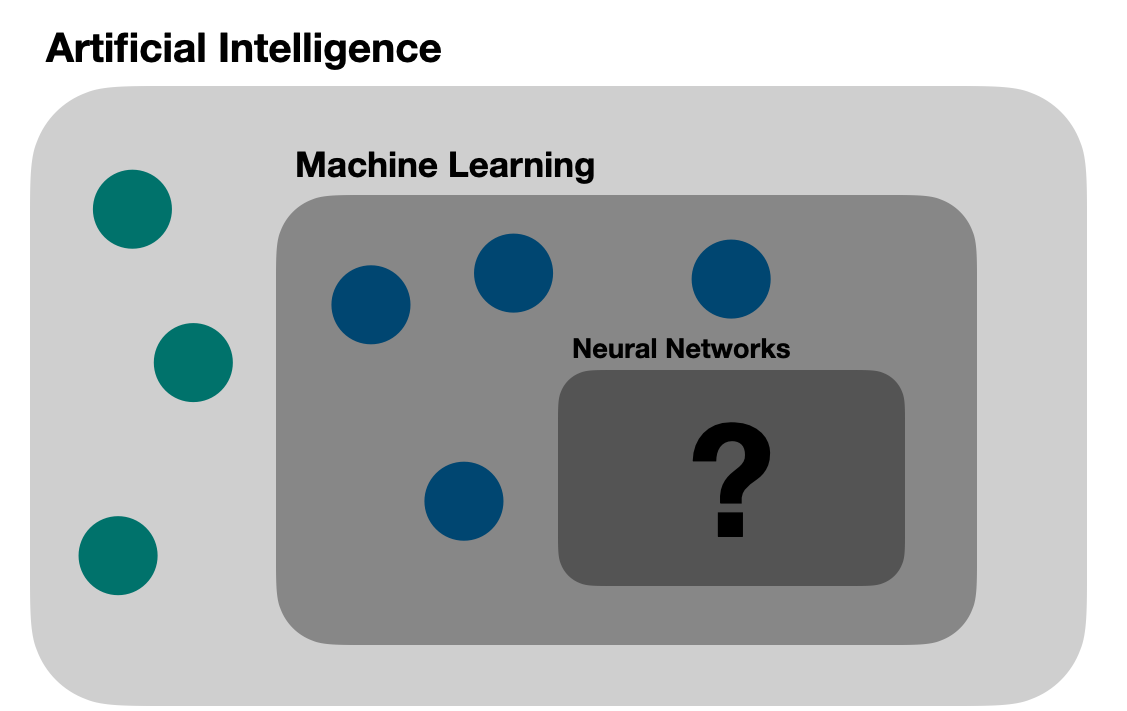## 📈 Linear and non-linear relationships

In mathematics, data relationships can be defined using a coordinate system with axes `x and y`.

Where these relationships exhibit linear proportionality, meaning the output can be predicted by following a linear proportion with the inputs, we refer to such relationships as linear.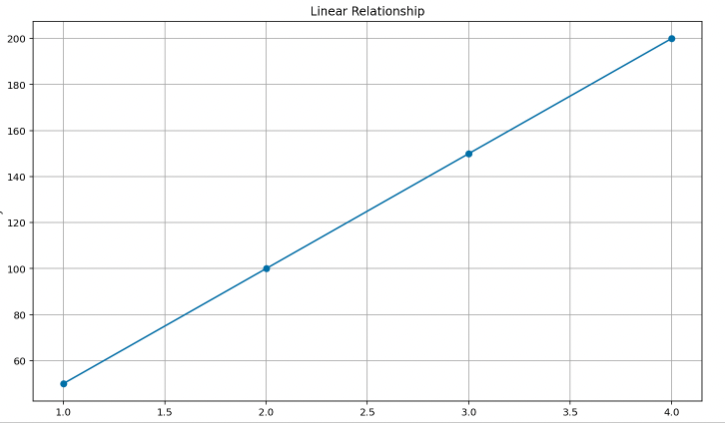Some types of linear relationships can be listed as follows:

• Linear regression

• Identify function

• Linear equations

• Proportional relationship

On the other hand, when the output cannot be predicted by following a linear proportion, meaning the line in the graph is not straight but curved instead, we classify to such relationships as non-linear.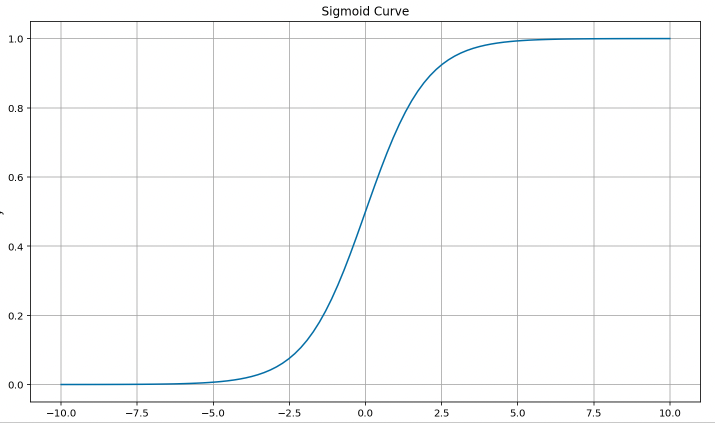Below, we can list some types of non-linear relationships:

• Logarithmic function

• Exponential function

• Sigmoid function

Now, time to go even further in this exciting journey into the realm of artificial neural networks.

## 🧠 An Overview of Artificial Neural Networks

To understand Artificial Neural Networks (ANN), it is important to gain insight into the functioning of the human brain.

### 🔵 It's all about neurons

The human brain is composed of billions of interconnected neurons, linked together by synaptic connections: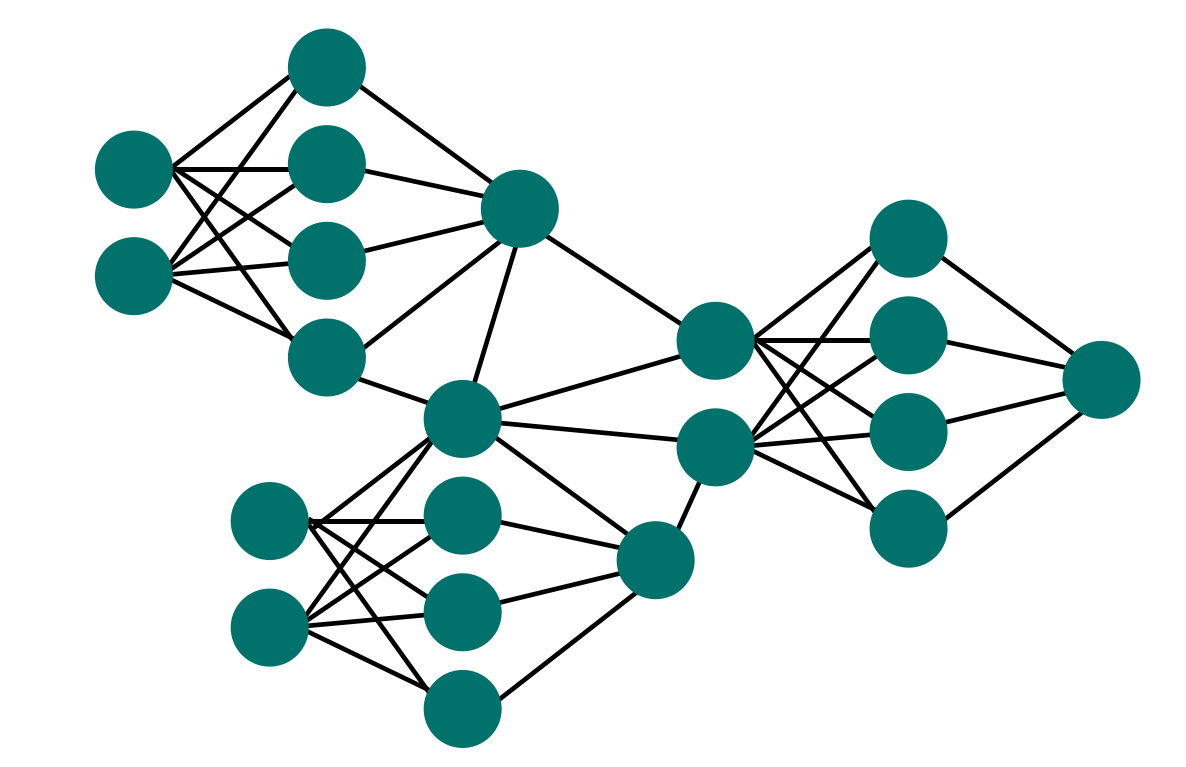Whenever our brain receives new information, it undergoes a process where the input is propagated forward through the interconnected neurons.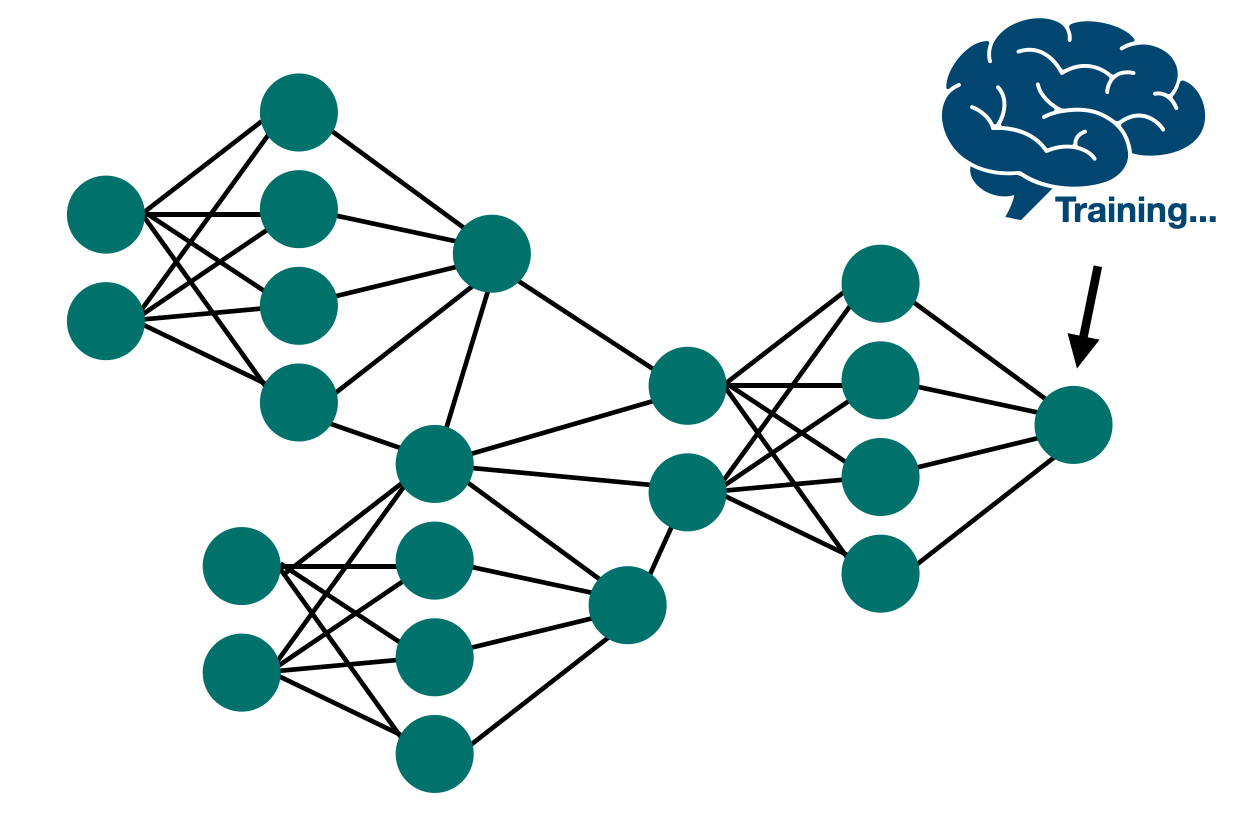Along the way, biases, acting as weights, are applied and propagated back, resulting in the generation of new knowledge.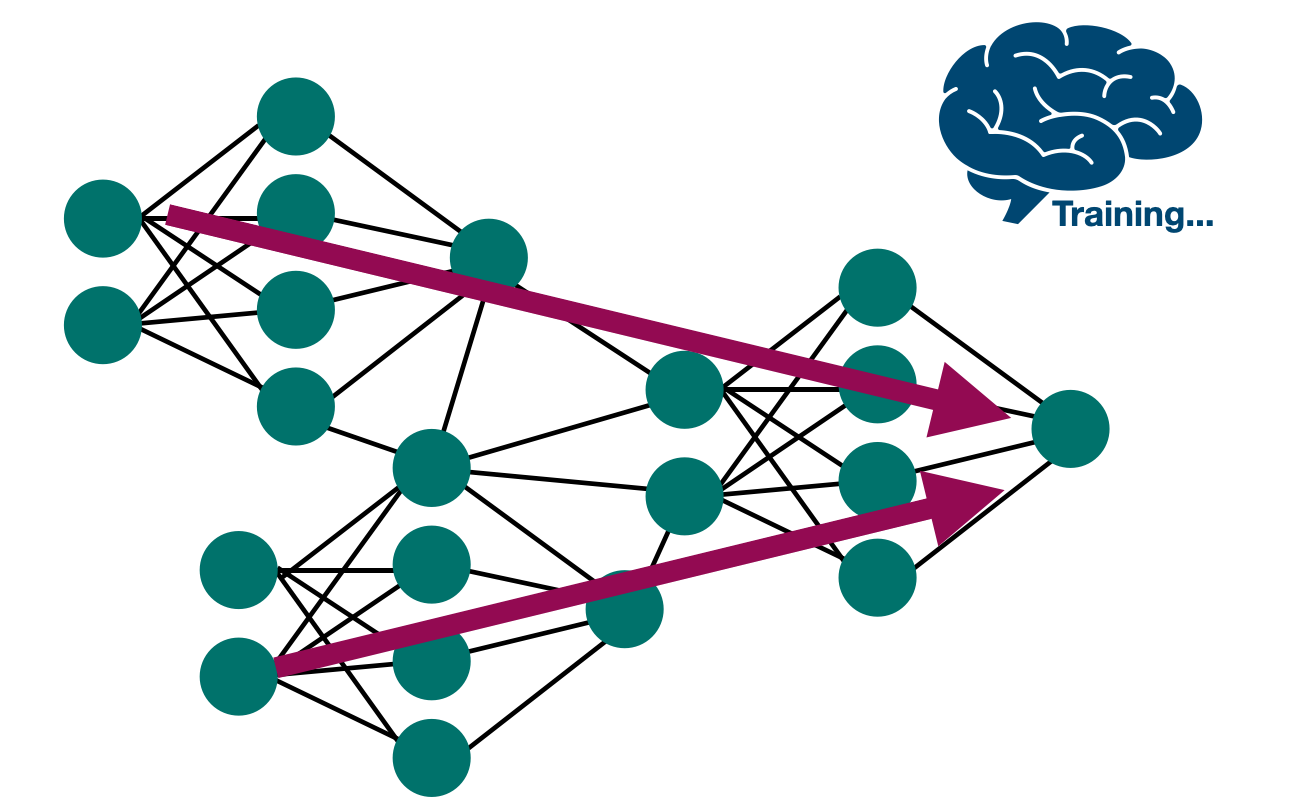This iterative process is known as learning or training.

## 🧠 Modeling the ANN

An ANN is essentially composed of multiple layers of interconnected neurons that contain weights or biases.

Similar to the human brain, ANNs follow a learning process that can be described as follows: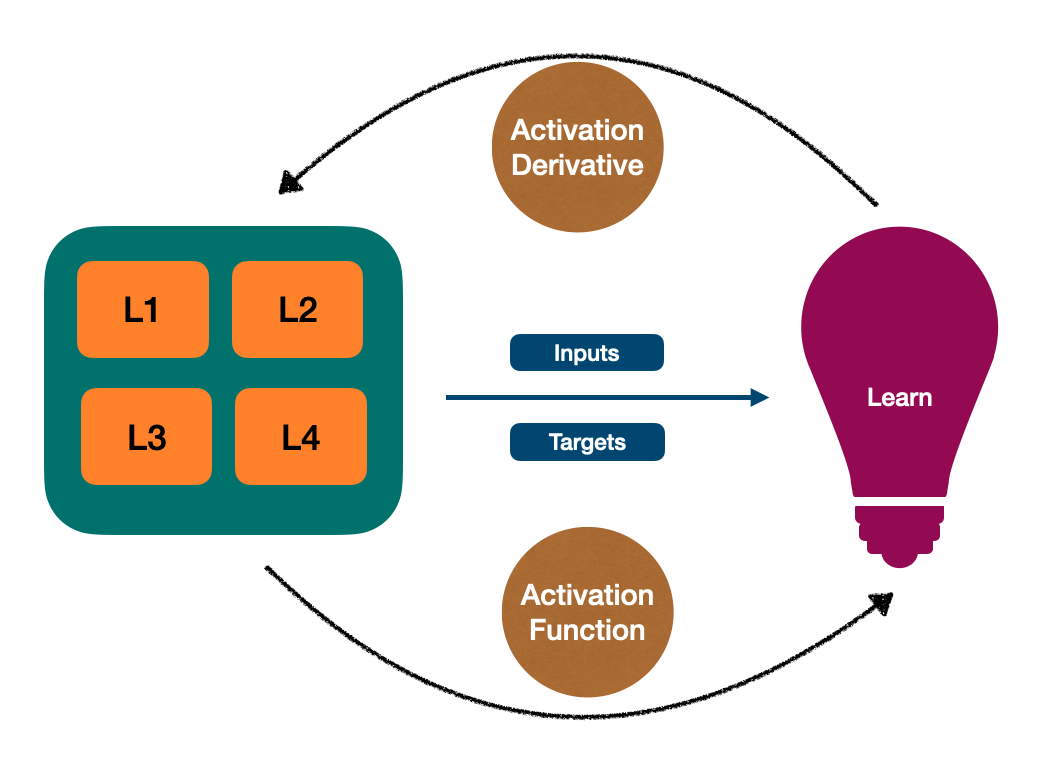• the inputs and targets are sent to the learning process

• an "activation function" is applied to the data going forward

• an "activation function derivative" is applied to the data being propagated back

💡 Wait... What is an activation function and its derivative?

👉 ANNs and data relationships

In machine learning, data can exhibit either linear or non-linear relationships.

Techniques like linear regression are suitable for capturing linear relationships, as they enable predictions based on the linear proportionality between inputs and outputs.

However, linear regression may not be an ideal choice for predicting non-linear data like pattern recognition. This is where other ML techniques, such as ANNs, come into play.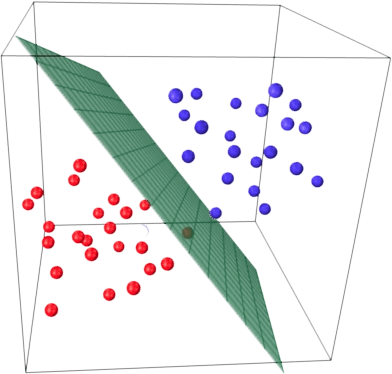👉 Activation function

Activation functions act like transforming inputs into non-linear relationships, allowing the creation of new knowledge.

ANNs offer a variety of non-linear functions that can be applied, including hyperbolic tangent, ReLU (Rectified Linear Unit), quadratic function, and sigmoid function, among others.

Each function brings unique properties and characteristics that make them suitable for different scenarios.

For the sake of simplicity in this article, we'll use the sigmoid function, also called the logistic function.

The sigmoid function is a mathematical function that takes a real number as input and converts it into another number ranging from 0 to 1.

It is characterized by an S-shaped curve, and its output value represents the probability or level of activation.When going propagated back, however, the derivative of the sigmoid function is applied. This derivative helps adjust the rate at which the resulting data deviates from the error margin.

``````class Calc
def self.sigmoid(number) = 1 / (1 + Math.exp(-number))
def self.sigmoid_derivative(number) = number * (1 - number)
end
``````

### 🔵 Layers & neurons

In Ruby, we can start by modelling the layers and neurons using a simple PORO object:

``````class Layer
attr_accessor :result

def initialize(neurons)
@neurons = neurons
end
end

###########################

class Neuron

def initialize(weights)
@weights = weights
end
end
``````

Next, we can define that our ANN is composed of 3 layers:

• an input layer consisting of 4 neurons, that contain 3 weights each

• a hidden layer consisting of 4 neurons, that contain 4 weights each

• and an output layer consisting of only 1 neuron, that contains 4 weights

``````# Input Layer (4 neurons -> 3 weights)
neuron_a = Neuron.new([-0.16595599, -0.70648822, -0.20646505])
neuron_b = Neuron.new([0.44064899, -0.81532281, 0.07763347])
neuron_c = Neuron.new([-0.99977125, -0.62747958, -0.16161097])
neuron_d = Neuron.new([-0.39533485, -0.30887855, 0.370439])
input_layer = Layer.new([neuron_a, neuron_b, neuron_c, neuron_d])

# Hidden Layer (4 neurons -> 4 weights)
neuron_e = Neuron.new([-0.16595599, -0.70648822, -0.20646505, -0.34093502])
neuron_f = Neuron.new([0.44064899, -0.81532281, 0.07763347, 0.44093502])
neuron_g = Neuron.new([-0.99977125, -0.62747958, -0.16161097, 0.14093502])
neuron_h = Neuron.new([-0.39533485, -0.30887855, 0.370439, -0.54093502])
hidden_layer = Layer.new([neuron_e, neuron_f, neuron_g, neuron_h])

# Output Layer (1 neuron -> 4 weights)
neuron_i = Neuron.new([-0.5910955, 0.75623487, -0.94522481, 0.64093502])
output_layer = Layer.new([neuron_i])
``````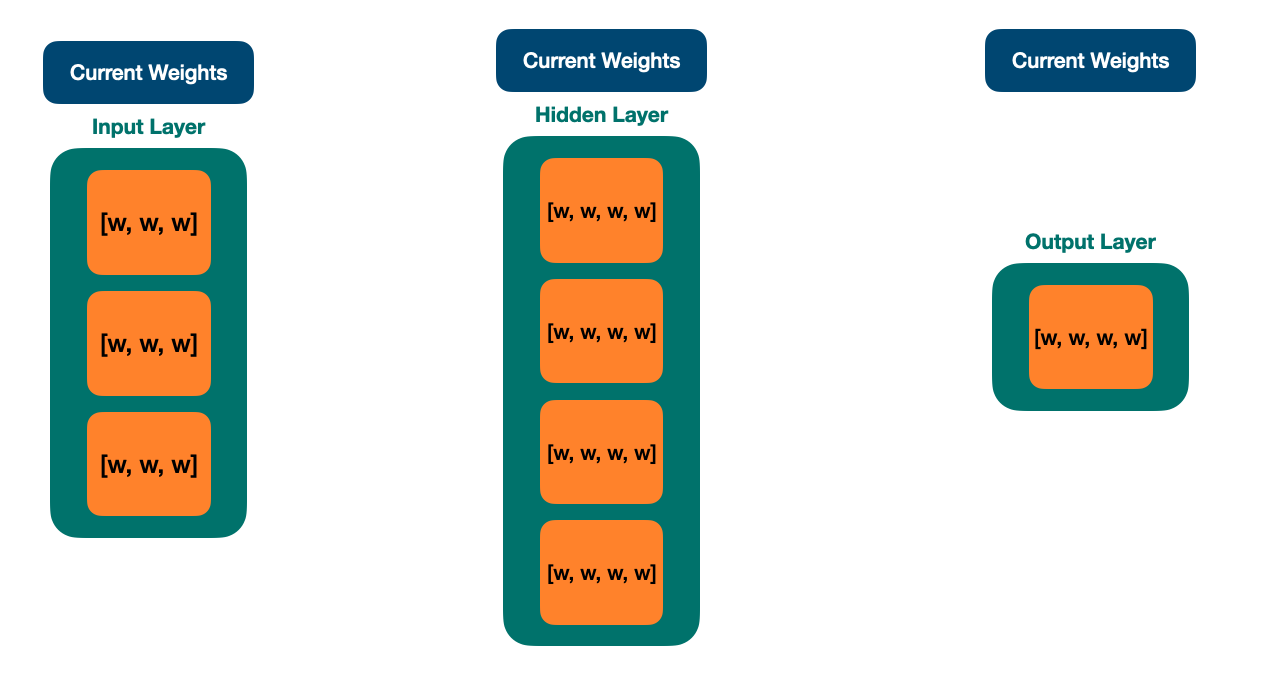### 🔵 Defining inputs and targets for an XOR gate

In this article, we're going to define that our ANN will predict the result of an XOR gate.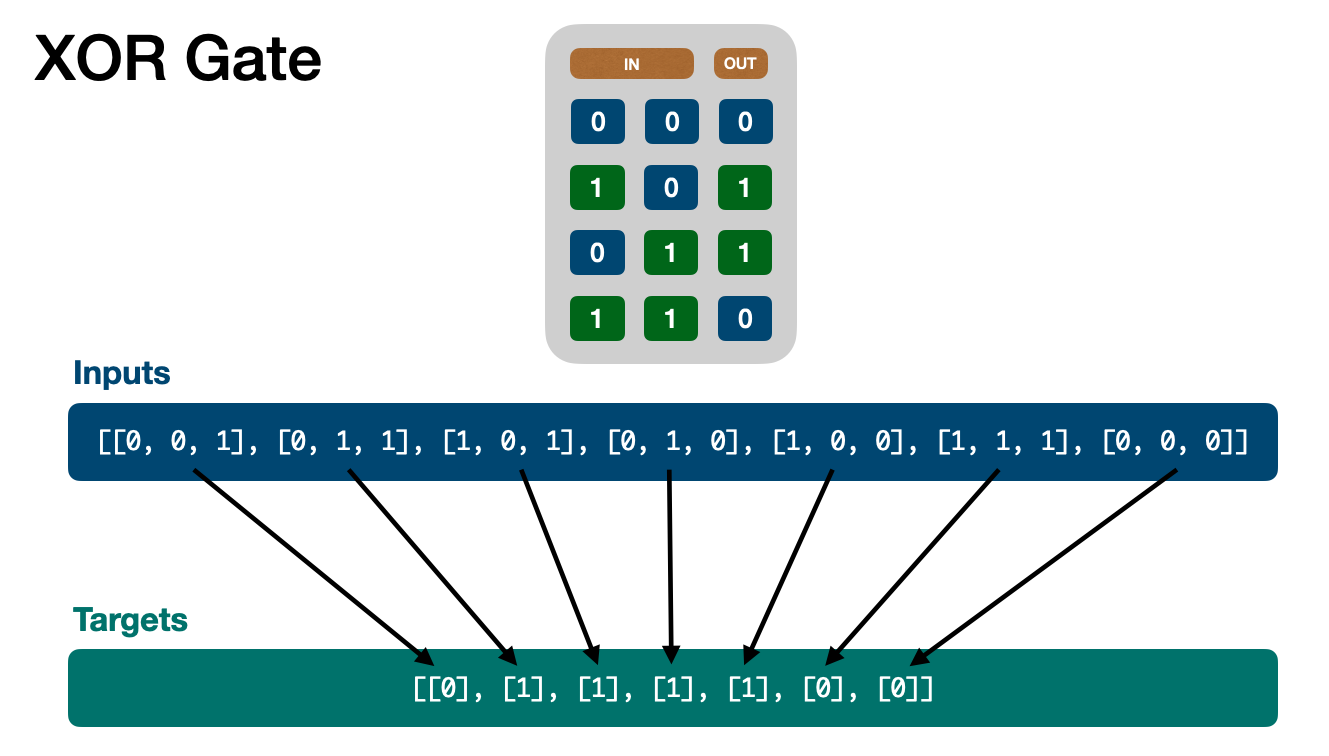``````inputs  = [[0, 0, 1], [0, 1, 1], [1, 0, 1], [0, 1, 0], [1, 0, 0], [1, 1, 1], [0, 0, 0]]
targets = [, , , , , , ]
``````

Due to the nature of being categorized as machine learning, which learns and makes predictions based on knowledge, an ANN essentially consists of two main processes:

• the learning process, which is the most important one

• the prediction process

ANN modelled, time to delve into the internal parts of the most important component: the learning process. Then, afterwards, we'll finish this article by explaining the prediction process.

## 🧠 The learning process

Based on the inputs and targets already modelled, we can define the ANN usage as follows:

``````network = NeuralNetwork.new([input_layer, hidden_layer, output_layer])

network.learn(inputs, targets, 2_000)
``````

Now, let's see the `learn` implementation:

``````class NeuralNetwork
def initialize(layers)
@layers = layers
end

def learn(inputs, targets, times)
times.times do
layers_with_results = ForwardPropagation.call(@layers, inputs)
@layers = BackPropagation.call(inputs, targets, layers_with_results)
end
end
end
``````
• First, the layers and inputs are sent to a component called `ForwardPropagation`, which returns the layers with calculated results for each layer

• Then, the layers with results are sent along with inputs and targets to another component called `BackPropagation`, which returns the layers adjusted, meaning that new knowledge has been created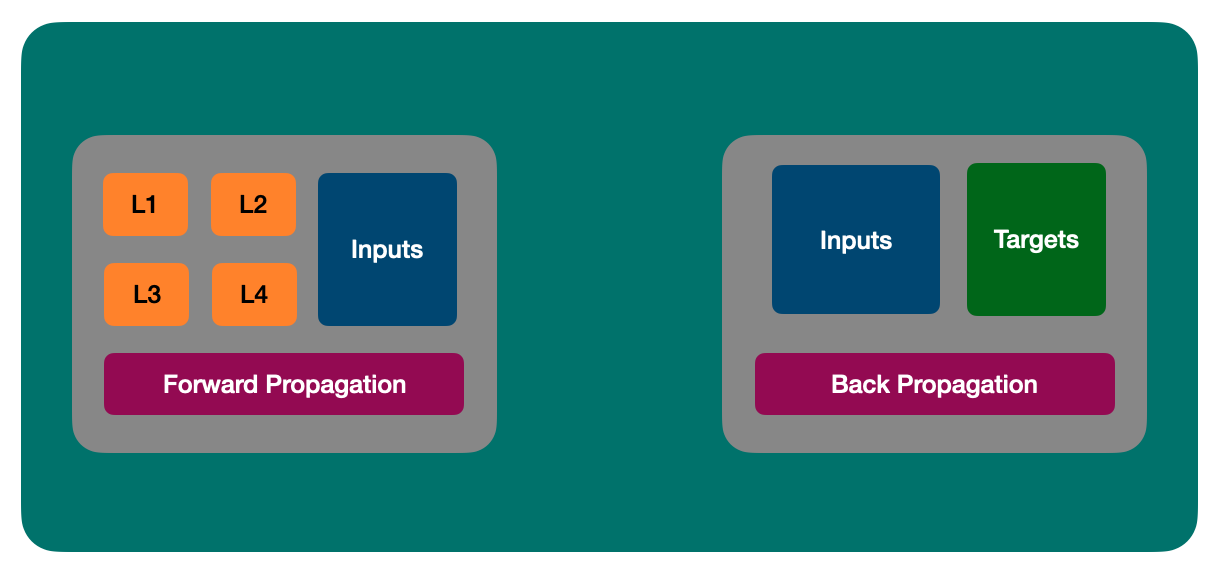💡 Be aware that the next parts will exhibit a lot of operations on matrices (multidimensional arrays). ANNs heavily rely on linear algebra during the learning process

## 🔵 Forward propagation

Forward propagation can be understood as the process in which the neural network takes inputs and passes them through each layer.

For every layer, the activation function (such as sigmoid) is applied to the multiplication between the received input and the biases (weights) associated with the current neuron.

This calculation is performed sequentially for each layer, allowing the network to generate output predictions based on the given inputs.

``````class ForwardPropagation
def self.call(*args) = new(*args).call

def initialize(layers, inputs)
@layers = layers.dup
@inputs = inputs
end

def call
@layers.map.with_index do |layer, index|
data = index.zero? ? @inputs : @layers[index - 1].result

layer.tap do
layer.result = (Matrix[*data] * Matrix[*layer.to_matrix]).map(&Calc.method(:sigmoid))
end
end
end
end
``````

And now, we can see the image representation of the forward propagation process: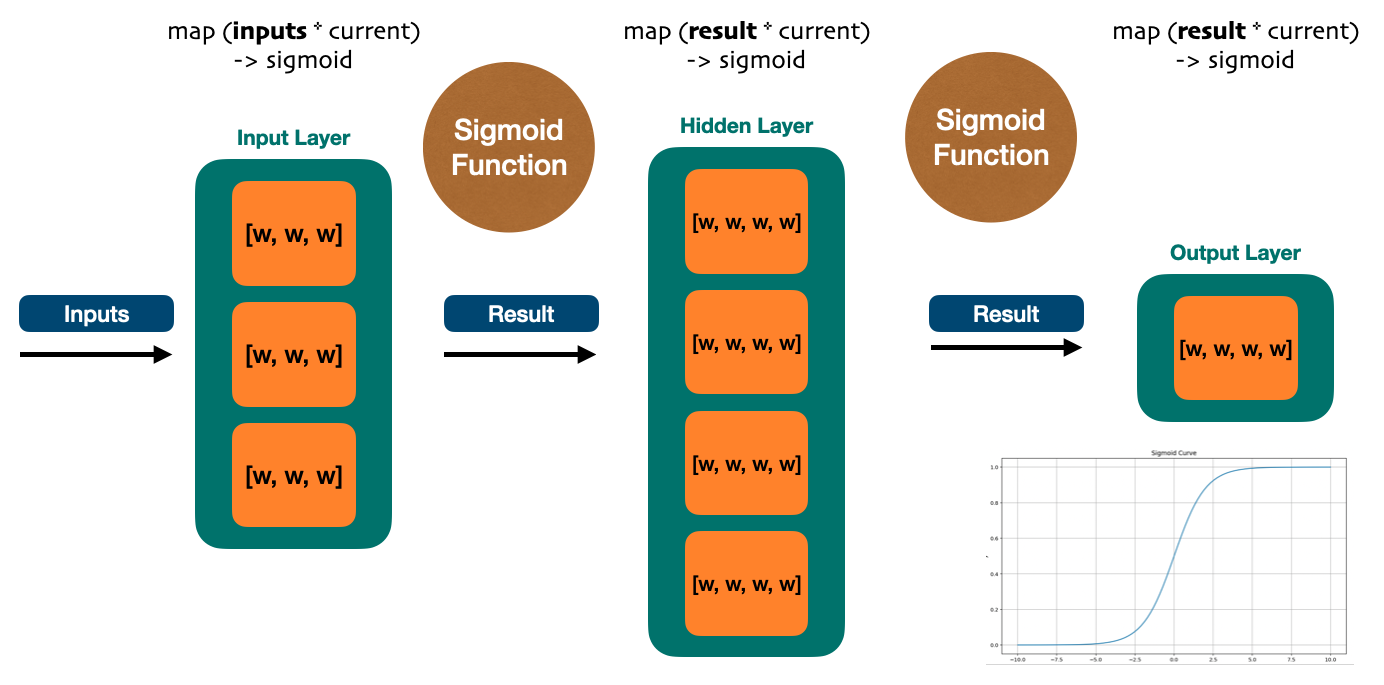After the forward propagation process, each layer has a new predicted result based on the non-linear normalization applied by the sigmoid function.

But we have to compare those results with the targets, calculate the error margin and lastly discover how far the result is from the desired output (targets).

You're correct, we are talking about backpropagation.

## 🔵 Backpropagation

Backpropagation is a crucial process where the predicted results from the forward propagation are recalculated for each layer.

This recalibration involves adjusting the weights and biases based on the calculated errors, to minimize the distance between the predicted results and the target values.

The entire process of forward and backpropagation is repeated iteratively until the predicted results closely match the target values, or until a desired level of accuracy is achieved.

This iterative repetition allows the neural network to continually improve its predictions by fine-tuning the weights and biases.

👉 Calculating deltas

Backpropagation starts by calculating deltas, which uses the `sigmoid function derivative` and exhibit how far the targets are from the error margin for each layer.

``````def apply_sigmoid_derivative(result)
result.to_a.map do |array|
array.map do |value|
Calc.sigmoid_derivative(value)
end
end
end

@layers.map.with_index do |layer, index|
result = index.zero? ? @inputs : previous_layer_of(layer).result

if layer == output_layer
error = Matrix[*@target] - Matrix[*output_layer.result]

NaiveMatrixMultiply.call(apply_sigmoid_derivative(output_layer.result).dup, error.to_a)
else
factor = output_layer.to_matrix.transpose
error = Matrix[*delta_output_layer] * Matrix[*factor]

NaiveMatrixMultiply.call(apply_sigmoid_derivative(layer.result).dup, error.to_a)
end
end
``````

• the error calculation may differ between layers

• the error for the output layer is calculated based on the target

• the error for the input and hidden layers is calculated based on the error of the output layer

• the sigmoid derivative is applied, which returns how far the target is from the error margin at the moment (delta)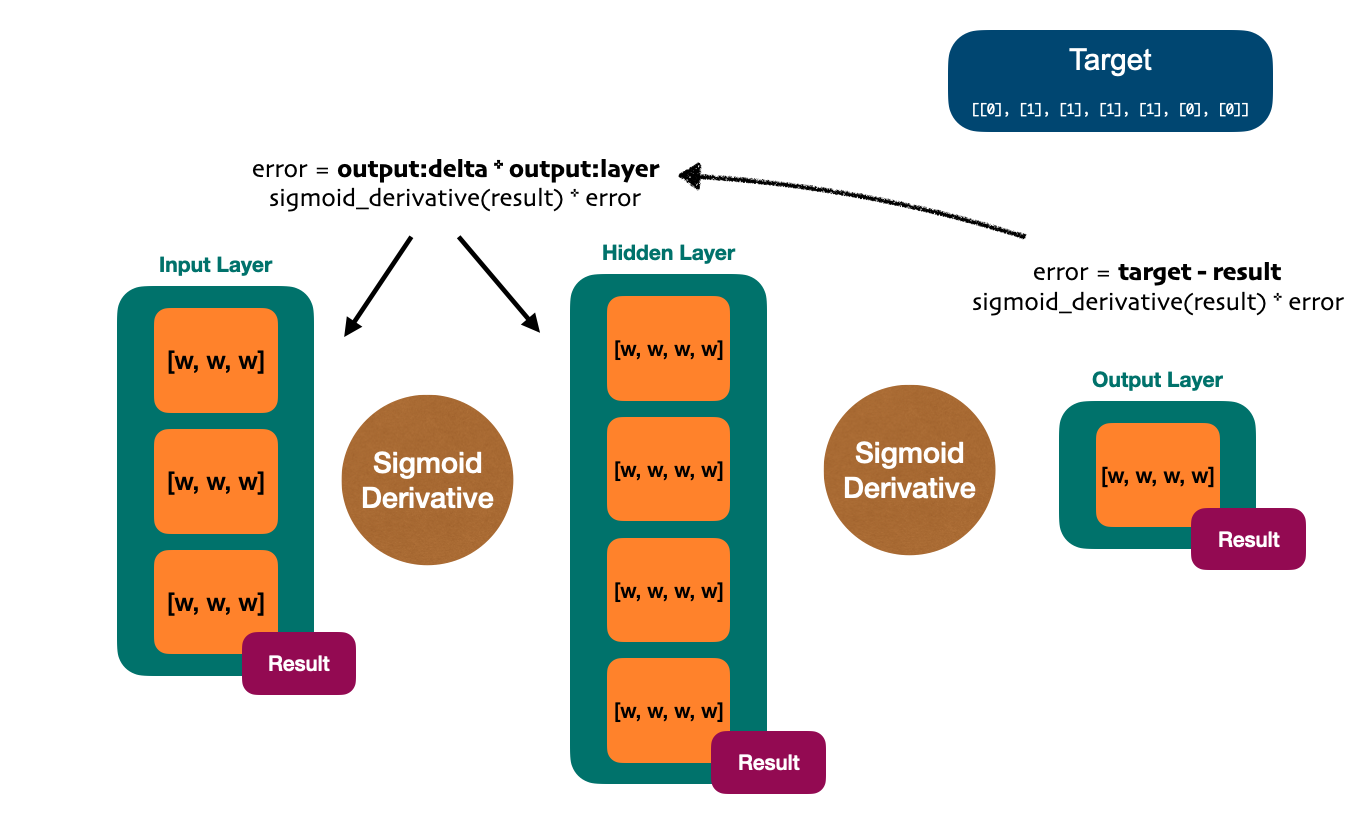After calculating the deltas during backpropagation, the process concludes by adjusting the weights (biases) of each interconnected neuron throughout all the layers in the ANN.

Leveraging the principles of linear algebra, these adjustments can be applied by performing matrix addition between the current weights matrix and the delta matrix, effectively updating the weights of the neurons in the network.

This matrix addition operation ensures that the adjustments are propagated through the network, enabling the network to learn and predict over time.

``````def adjusted_layer(layer, index)
result = index.zero? ? @inputs : previous_layer_of(layer).result
delta = delta(layer)

end
``````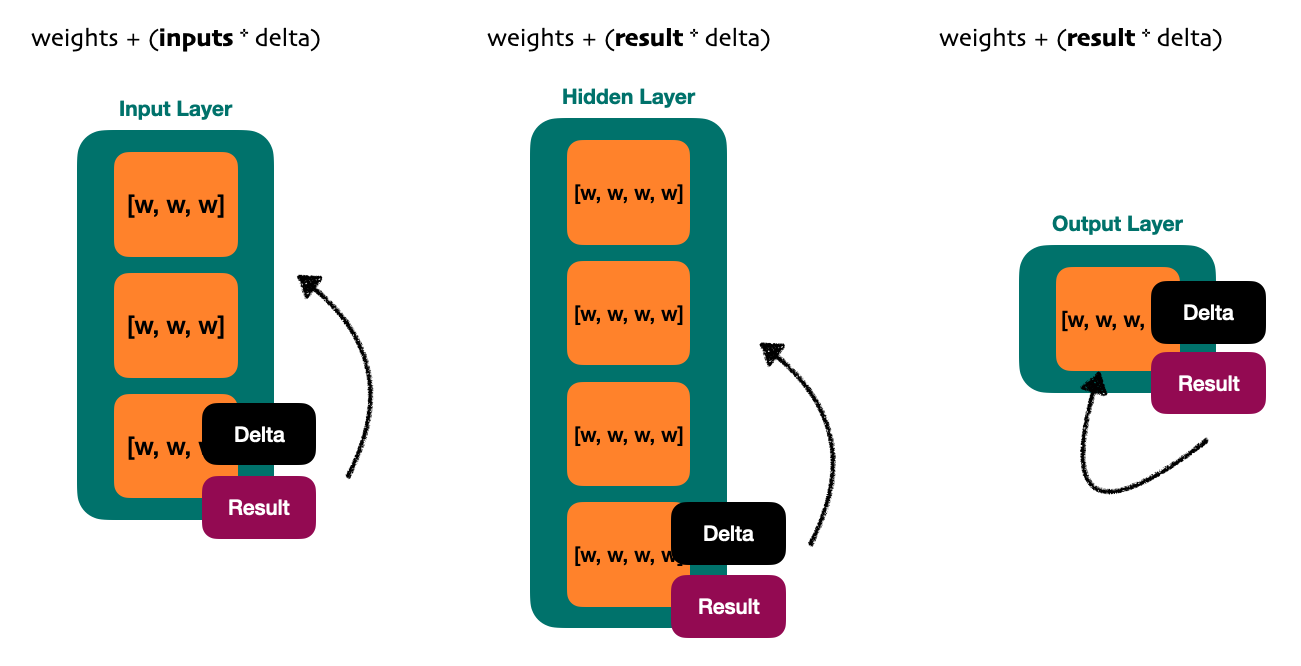The following image describes an overview of backpropagation process: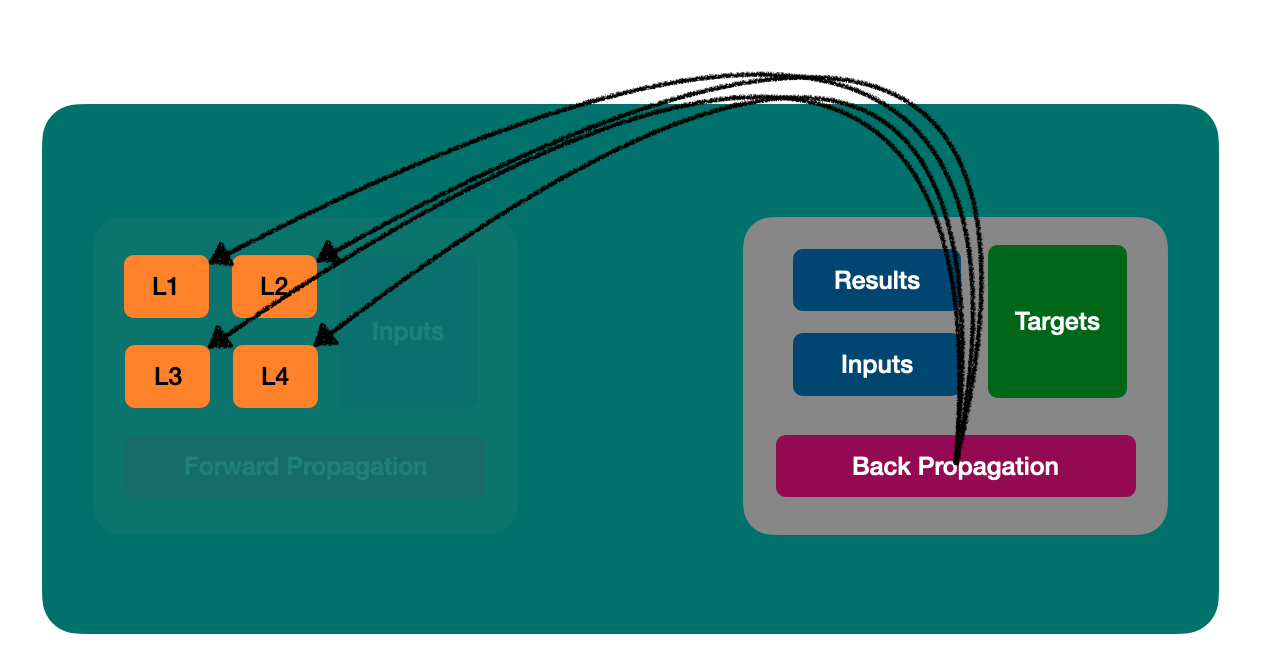In the below image representation, we can visualize an overview of the entire learning process: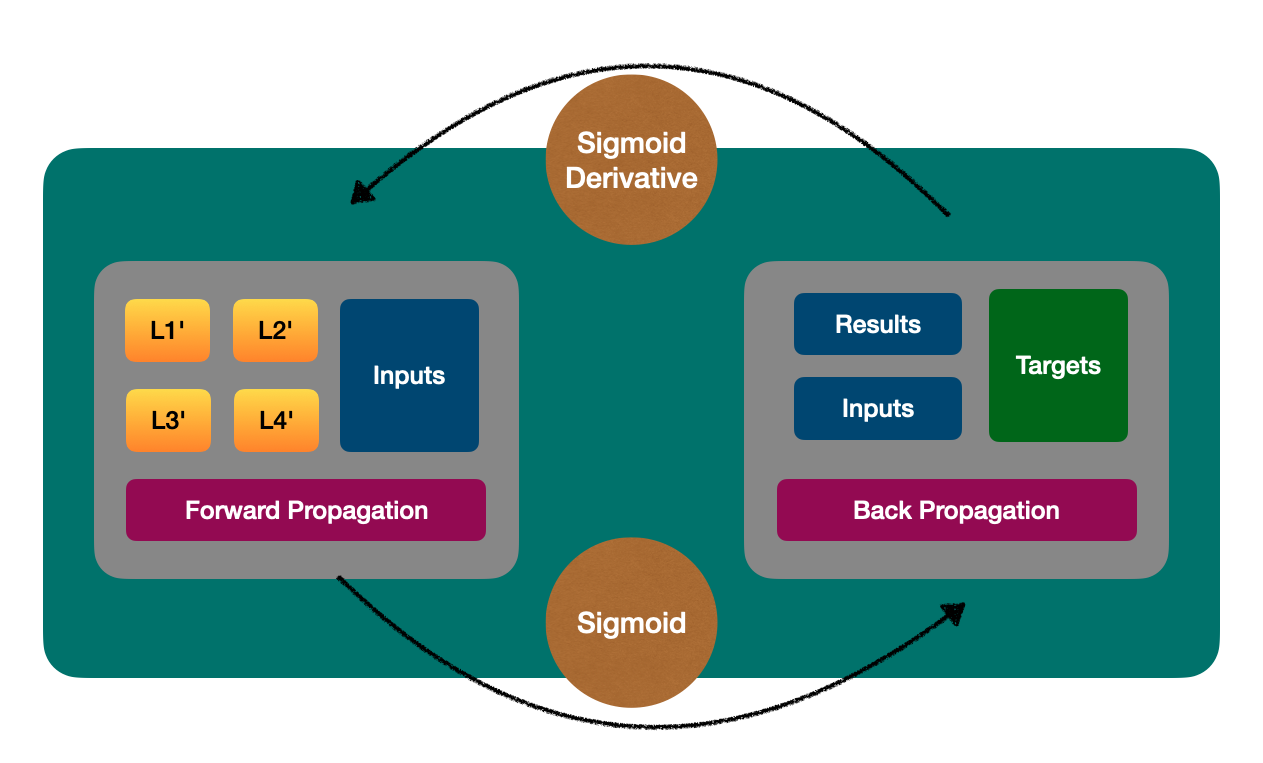💡 Our ANN can learn, but can it make predictions?

Simply using the forward propagation process.

## 🧠 The prediction process

The prediction process is indeed simpler compared to the learning process.

During prediction, we only need to retrieve the predicted results from the output layer, which is the final layer of the network, obtained during the forward propagation.

This allows us to quickly obtain the model's predictions for a given input without the need for the extensive calculations involved in the learning process.

It is simple as doing:

``````class Predict
def self.call(*args) = new(*args).call

def initialize(layers, inputs)
@layers = layers.dup
@inputs = inputs
end

def call
layers_with_results = ForwardPropagation.call(@layers, @inputs)
output_layer_result = layers_with_results.last.result

output_layer_result.first
end
end
``````

If the learning process can be described as follows: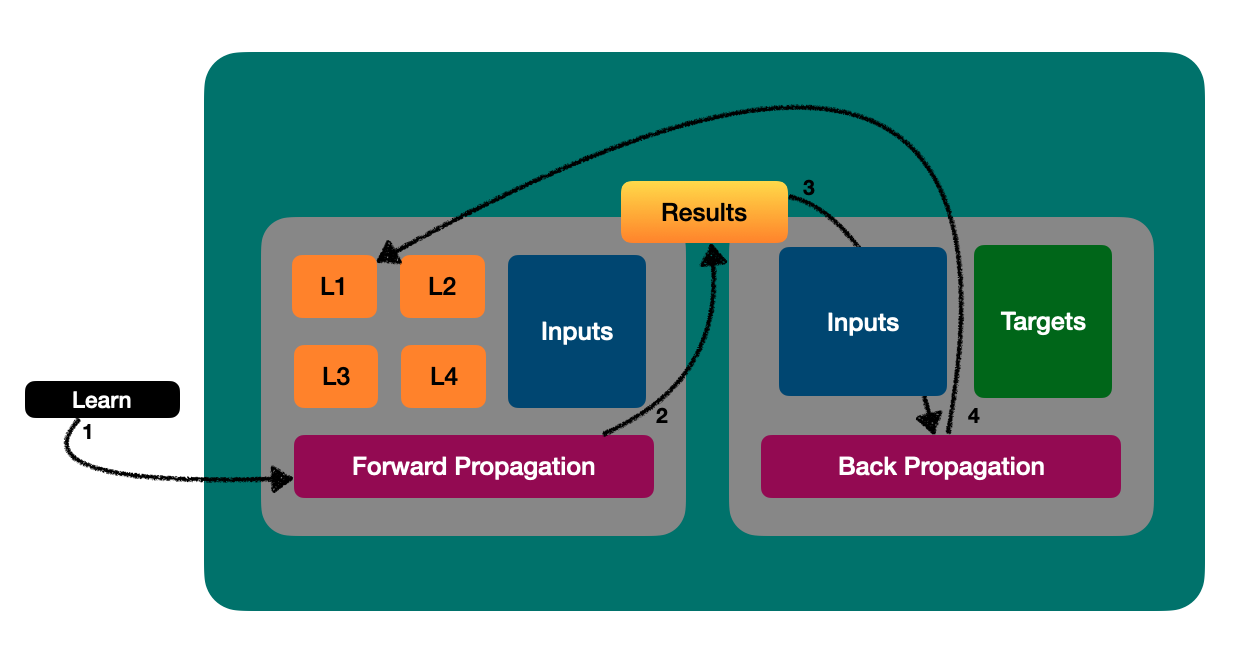Then the prediction process gets the following representation: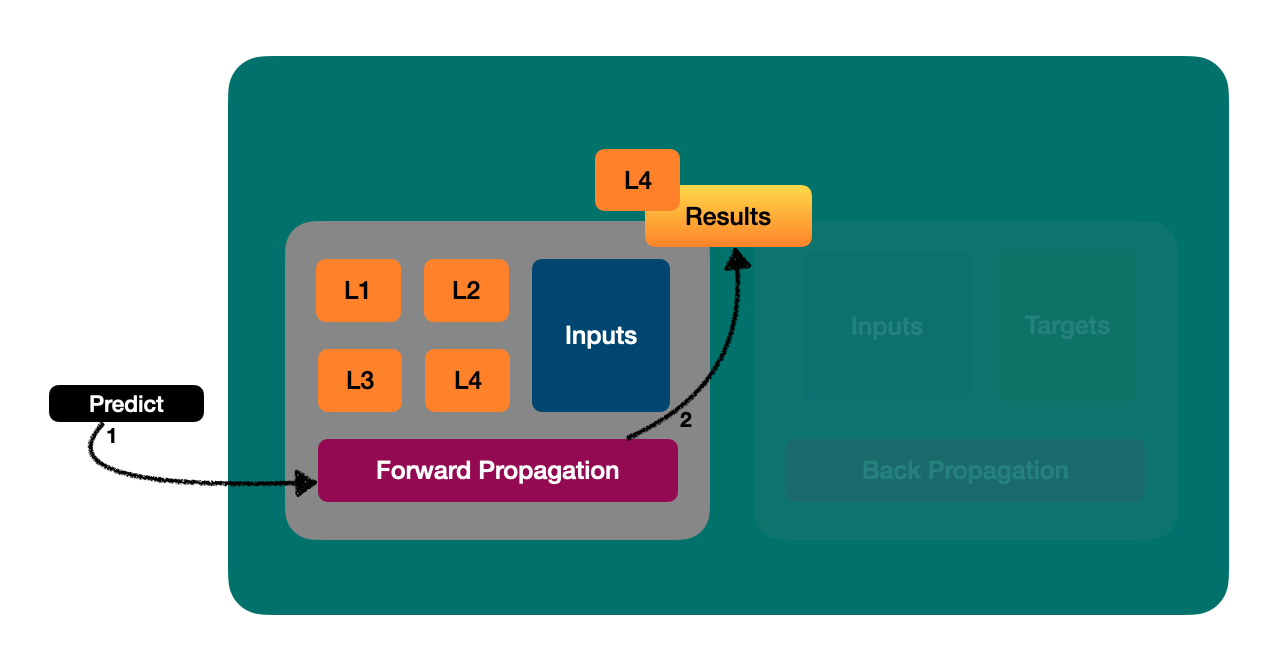Yay!

## 🧠 Some test cases in Ruby

In the following sections, there are two examples of test cases in Ruby, showing the capabilities of the ANN built in this article.

👉 Predicting XOR gate

``````class TrainXORTest < Test::Unit::TestCase
def test_train_xor_gate
# Setup...

inputs  = [[0, 0, 1], [0, 1, 1], [1, 0, 1], [0, 1, 0], [1, 0, 0], [1, 1, 1], [0, 0, 0]]
targets = Matrix[[0, 1, 1, 1, 1, 0, 0]].transpose.to_a

network = NeuralNetwork.new([layer_a, layer_b, layer_c])
network.learn(inputs, targets, 2_000)

assert_equal 0.05, network.predict([[1, 1, 0]]).round(2)
end
end
``````

👉 Predicting fruits and vegetables

``````class TrainFruitsTest < Test::Unit::TestCase
def test_train_fruits_and_vegetables
# Setup...

# Prepare data model
fruits_func = -> do
[(1..3).to_a.shuffle.take(3), 0]
end

vegetables_func = -> do
[(7..9).to_a.shuffle.take(3), 1]
end

fruits_inputs = 50.times.map { fruits_func.call }
vegetables_inputs = 50.times.map { vegetables_func.call }
inputs = fruits_inputs.to_h.keys + vegetables_inputs.to_h.keys
outputs = fruits_inputs.to_h.values + vegetables_inputs.to_h.values
targets = Matrix[outputs].transpose.to_a

network = NeuralNetwork.new([layer_a, layer_b, layer_c])
network.learn(inputs, targets, 2_000)

fruits_and_vegetables = [
['Apple', fruits_func.call],
['Banana', fruits_func.call],
['Carrot', vegetables_func.call],
['Orange', fruits_func.call],
['Tomato', vegetables_func.call],
['Pineapple', fruits_func.call],
['Potato', vegetables_func.call],
['Cherry', fruits_func.call],
['Garlic', vegetables_func.call],
['Broccoli', vegetables_func.call],
['Peach', fruits_func.call],
['Pear', fruits_func.call],
['Lettuce', vegetables_func.call]
]

fruits = %w[Apple Banana Orange Pineapple Cherry Peach Pear]
vegetables = %w[Carrot Tomato Potato Garlic Broccoli Lettuce]

fruits.each do |fruit|
assert network.predict([fruits_and_vegetables.to_h[fruit]]) < 0.5
end

vegetables.each do |vegetable|
assert network.predict([fruits_and_vegetables.to_h[vegetable]]) > 0.95
end
end
end
``````

## Wrapping Up

In this article, we have covered the fundamental concepts of artificial intelligence, machine learning, and neural networks, exploring how they are related.

Additionally, we delved into the inner workings of a neural network while building a simple ANN that is capable of predicting things like XOR gate and fruits/vegetables using Ruby.

You can find all the code written in this article in my ANN project called citrine. I have an ANN written in Elixir too. Feel free to fork, clone, and experiment with the fascinating world of ANNs on your own.

## References

https://stevenmiller888.github.io/mind-how-to-build-a-neural-network/

https://medium.com/technology-invention-and-more/how-to-build-a-simple-neural-network-in-9-lines-of-python-code-cc8f23647ca1#.voyy4g51x